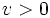# Finiteness theorem

Let$n \in \mathbb{N}$,$\Lambda < \infty$,$D < \infty$m and$v > 0$. Then consider the space$S$ of differential manifolds$M$ admitting a metric$g$ for which:$dim(M) = n, |K| \le \Lambda, diam(M) \le D, vol(M) \ge v$
where$K$ denotes the sectional curvature.
Then,$S$ consists of finitely many diffeomorphism classes/types.